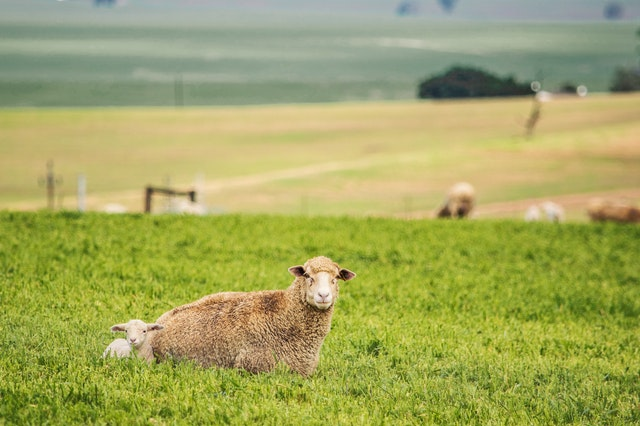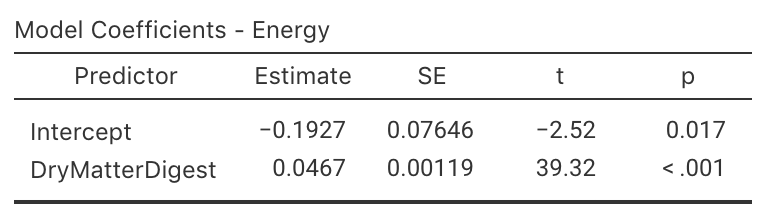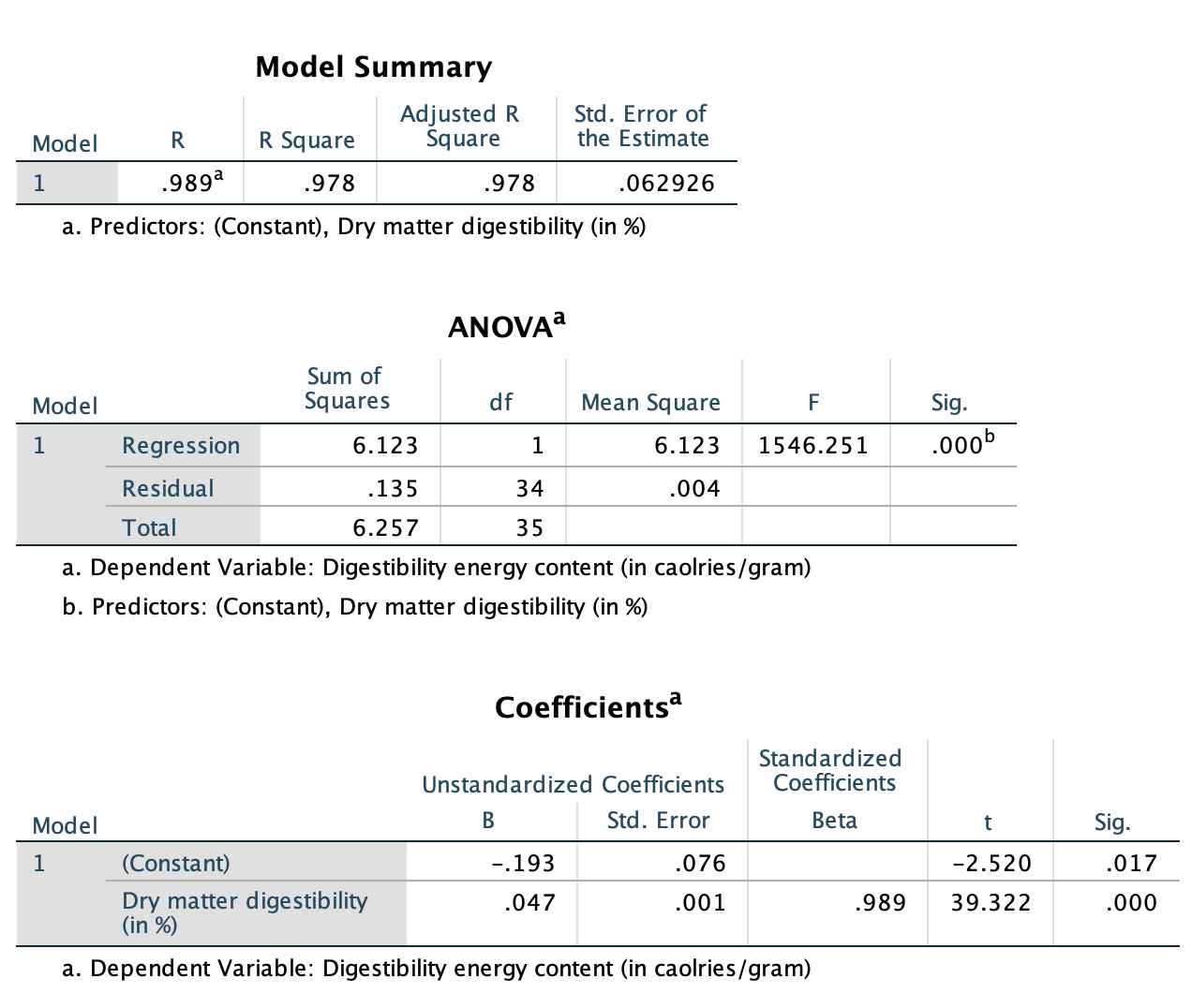## 35.10 Example: Food digestibilityA study evaluated various food for sheep . One combination of variables assessed is shown in Fig. 33.6.

The RQ is:

Does the digestible energy requirement of feed increase with dry matter digestibility percentage (and if so, what is the relationship)?

In this study, $$x$$ is the dry matter weight digestibility percentage, and $$y$$ is the digestible energy. The data are shown in Fig. 35.16. Using the software output (Fig. 35.17 (jamovi); Fig. 35.18 (SPSS)), the values of the slope and $$y$$-intercept in the sample are $$b_0 = -0.193$$ and $$b_1 = 0.047$$. The regression equation is

$\hat{y} = -0.193 + 0.047x.$

FIGURE 35.16: The digestibility data set

The slope means that when the dry matter weight digestibility increases by 1 percentage point, the digestible energy increases, on average, by 0.047 Cal/gram.

Each sample will produce slightly different sample slopes, so we can test to see if the slope in the population is non-zero due to sampling variation, using a hypothesis test:

• $$H_0$$: $$\beta_1 = 0$$;
• $$H_1$$: $$\beta_1 > 0$$.

The parameter is $$\beta_1$$, the population slope for the regression equation predicting digestible energy from dry matter weight.

The alternative hypothesis is one-tailed, based on the RQ.

From the software output, $$t = 39.322$$, which is huge; the two-tailed $$P$$-value is $$P<0.001$$. Since we have a one-tailed alternative hypothesis, the $$P$$-value is less than $$0.001/2 = 0.0005$$. There is very strong evidence that the digestible energy increases as the dry matter weight digestibility increases.

The approximate 95% CI for the population slope $$\beta_1$$ is

$0.047 \pm (2\times 0.001),$ or from 0.045 to 0.049.FIGURE 35.17: jamovi output for the sheep-feed dataFIGURE 35.18: SPSS output for the sheep-feed data

The results are statistically valid. We write:

The sample presents very strong evidence ($$t = 39.322$$; one-tailed $$P < 0.0005$$) of a relationship between dry matter weight digestibility and the digestible energy (slope: $$0.047$$; $$n = 36$$; 95% CI from $$-0.045$$ to $$-0.049$$) in the population.

Click on the hotspots in the following image, to see what the areas under the normal curve mean.

### References

Moir RJ. A note on the relationship between the digestible dry matter and the digestable energy content of ruminant diets. Australian Journal of Experimental Agriculture and Animal Husbandry. 1961;1:24–6.